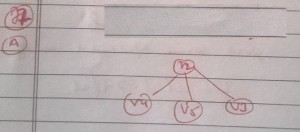Open in App
Not now

# GATE | Gate IT 2008 | Question 74

• Last Updated : 28 Jun, 2021

A binary tree with n > 1 nodes has n1, n2 and n3 nodes of degree one, two and three respectively. The degree of a node is defined as the number of its neighbors.

Starting with the above tree, while there remains a node v of degree two in the tree, add an edge between the two neighbors of v and then remove v from the tree. How many edges will remain at the end of the process?
(A) 2 * n1 – 3
(B) n2 + 2 * n1 – 2
(C) n3 – n2
(D) n2 + n1 – 2